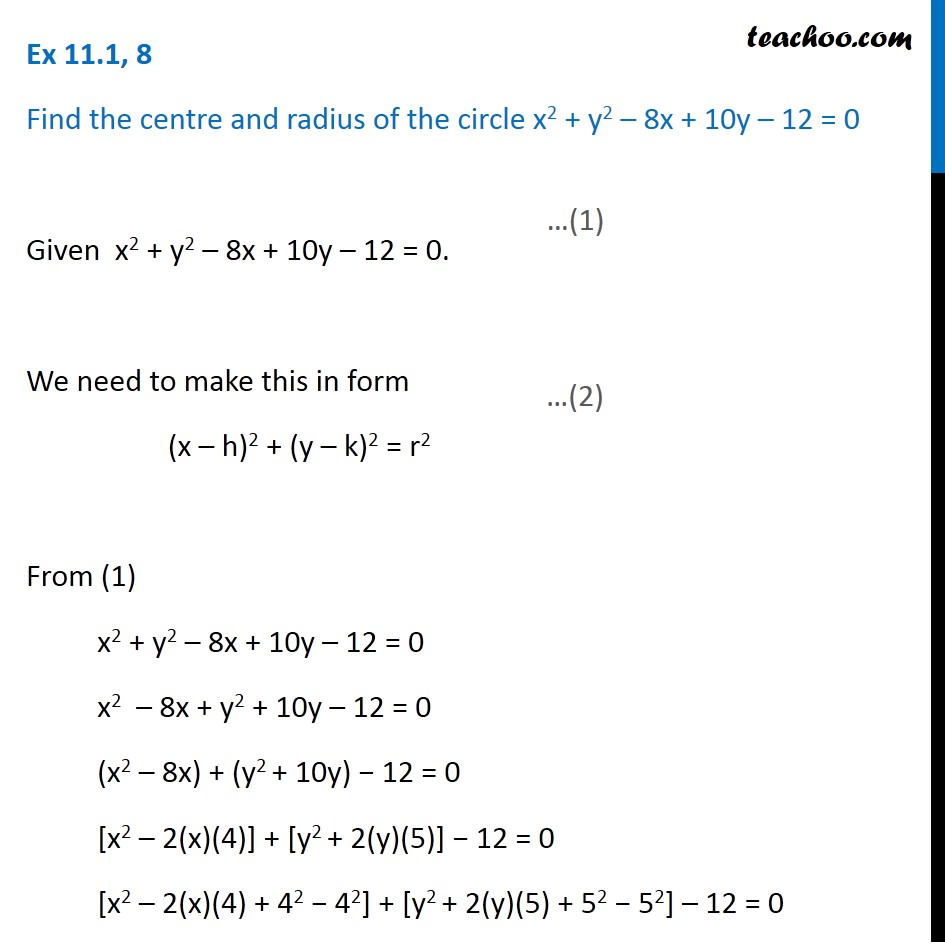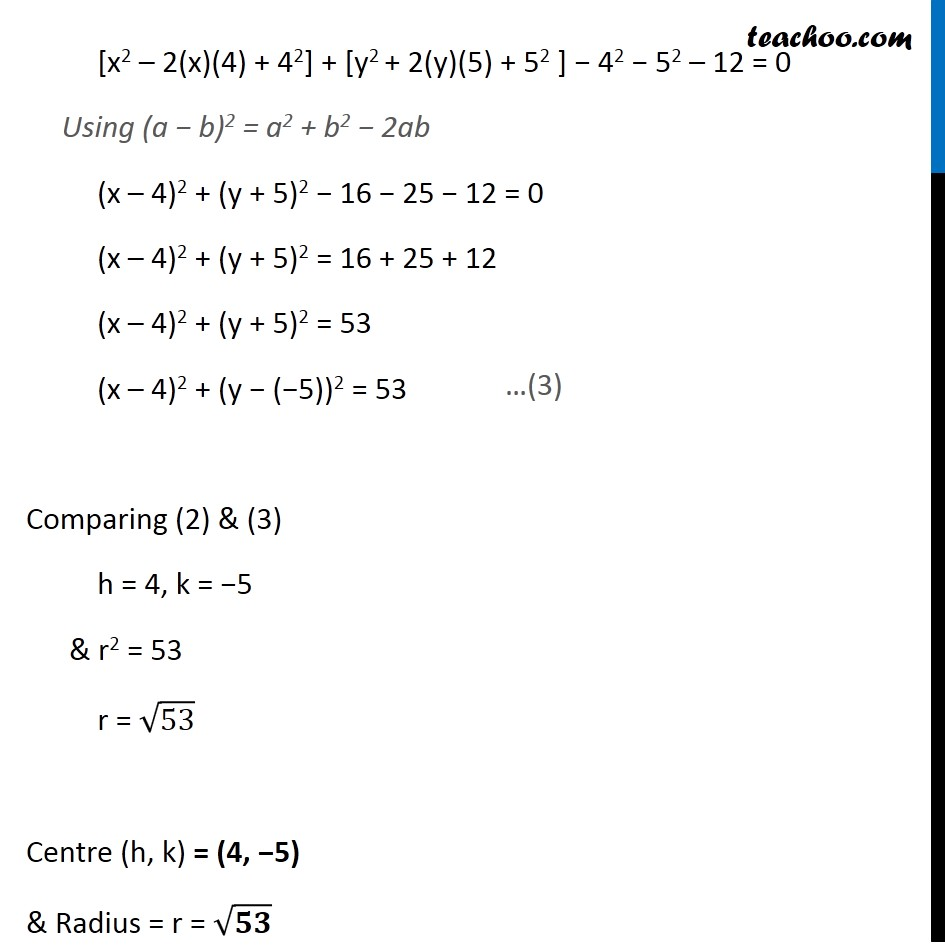Circle

Chapter 11 Class 11 Conic Sections
Concept wiseIntroducing your new favourite teacher - Teachoo Black, at only ₹83 per month

### Transcript

Ex 11.1, 8 Find the centre and radius of the circle x2 + y2 – 8x + 10y – 12 = 0 Given x2 + y2 – 8x + 10y – 12 = 0. We need to make this in form (x – h)2 + (y – k)2 = r2 From (1) x2 + y2 – 8x + 10y – 12 = 0 x2 – 8x + y2 + 10y – 12 = 0 (x2 – 8x) + (y2 + 10y) − 12 = 0 [x2 – 2(x)(4)] + [y2 + 2(y)(5)] − 12 = 0 [x2 – 2(x)(4) + 42 − 42] + [y2 + 2(y)(5) + 52 − 52] – 12 = 0 [x2 – 2(x)(4) + 42] + [y2 + 2(y)(5) + 52 ] − 42 − 52 – 12 = 0 Using (a − b)2 = a2 + b2 − 2ab (x – 4)2 + (y + 5)2 − 16 − 25 − 12 = 0 (x – 4)2 + (y + 5)2 = 16 + 25 + 12 (x – 4)2 + (y + 5)2 = 53 (x – 4)2 + (y − (−5))2 = 53 Comparing (2) & (3) h = 4, k = −5 & r2 = 53 r = √53 Centre (h, k) = (4, −5) & Radius = r = √𝟓𝟑